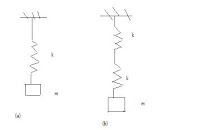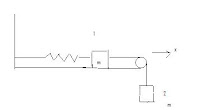# Objective Question for SHM(Simple Harmonic Motion)

Q 1. Total energy of mass spring system in harmonic motion is E=1/2(m&#9692A2). Consider another system executing SHM with same amplitude having value of spring constant as half the previous one and mass twice as that of previous one. The energy of second oscillator will be

(a) E
(b) 2E
(c) &#8730 2E
(d) E/2

Q 2. A particle is executing linear SHM of amplitude A. What fraction of total energy is potential when the displacement is 1/4 times amplitude.

(a) 3/2
(b) 1/16
(c) 1/4
(d) 1/2&#8730 2

Q 3. Fig below shows two spring mass systems. All the springs are identical having spring constant k and are of negligible mass. If m is the mass of block attached to the spring then the ratio of time period of oscillations of both systems is(a) 1:2&#8730 2
(b) 1:1/2&#8730 2
(c) 1:&#8730 2
(d) &#8730 2:1

Q 4. Fig below shows two equal masses of mass m joined by a rope passing over a light pulley. First mass is attached to a spring and another end of spring is attached to a rigid support. Neglecting frictional forces total energy of the system when spring is extended by a distance x is(a) mv2+1/2(Kx2)+mgx
(b) mv2-1/2(Kx2)+mgx
(c) mv2-1/2(Kx2)-mgx
(d) mv2+1/2(Kx2)-mgx

where v = dx/dt , the velocity of mass

Q 5. A spring of force constant k is cut into two pieces such that one piece is four times the length of the other. the longer piece will have force constant equal to

(a) 4k/5
(b) 5k/4
(c) 3k/2
(d) 4k

Q 6. In the system shown below frequency of oscillation when mass is displaced slightely is(a) f=1/2&#960(k1k2/(k1+k2)m)1/2
(b) f=1/2&#960((k1+k2)/m)1/2
(c) f=1/2&#960(m/(k1k2))1/2
(d) f=1/2&#960((k1+k2)/(k1k2)m)1/2

Q 7. A simple pendulum is displaced from it's mean position o to a position A such that height of A above O is 0.05m. It is then released it's velocity when it passes mean position is

(a) .1m/s
(b) 5.0m/s
(c) 1m/s
(d) 1.5m/s

Q 8. A particle is executing SHM at mid point of mean position and extreme position . What is it's KE in terms of total energy E.

(a) E/2
(b) 4E/3
(c) &#8730 2E
(d) 3E/4

Q 9. A solid cylinder of radius r and mass m is connected to a spring of spring constant k and it slips on a friction less surface without rolling with angular frequency(a) &#8730(k/mr)
(b) &#8730(kr/m)
(c) &#8730(k/m)
(d) &#8730(2k/m)

Solutions
1. (d)
Hint Substitute the values in energy equation

2. (b)
Hint
PE=(1/2)mw2x2
E=(1/2)mw2A2

3.(c)
Hint
T=2&#960√m/K
For first case
K'=K
For second case
K'=K/2

4.(d)
For mass at position 1 and spring system
KE=1/2mv2
PE=1/2kx2
For mass at position 2
KE=1/2mv2
PE=-mgx
Total energy of the system
E=mv2+1/2kx2-mgx

5.(b)

6.(a)
Hint parallel connection of two capacitors is electrical analogue

7.(c)
Hint
v=√2gh

8.(d)

9. (c)